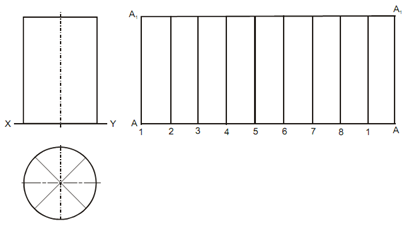## Development of the Surface of a Right Cylinder Assignment Help

Assignment Help: >> Development of a Pyramid - Development of the Surface of a Right Cylinder

Development of the Surface of a Right Cylinder:

Procedure

(a) Sketch a plan and elevation of a cylinder with the given dimensions.

(b) Divide a circle or of the plan in a number of equal parts as like 8 by sketching diameters. After that project these divisions in the elevation. All line in the elevation represents a generator.(c) Sketch horizontal lines on the side of the elevation. These lines are named as stretch-out lines as like A A and A1, A1. The length of these lines is the same to the circumference of the cylinder p × D, where D is the diameter of the cylinder.

(d) Divide the stretch-out line into the equal number of same parts whether the plan circle has been divided or here, eight parts.

(e) The rectangle (A A A1 A1), so obtained, is the development of the lateral surface of the cylinder.